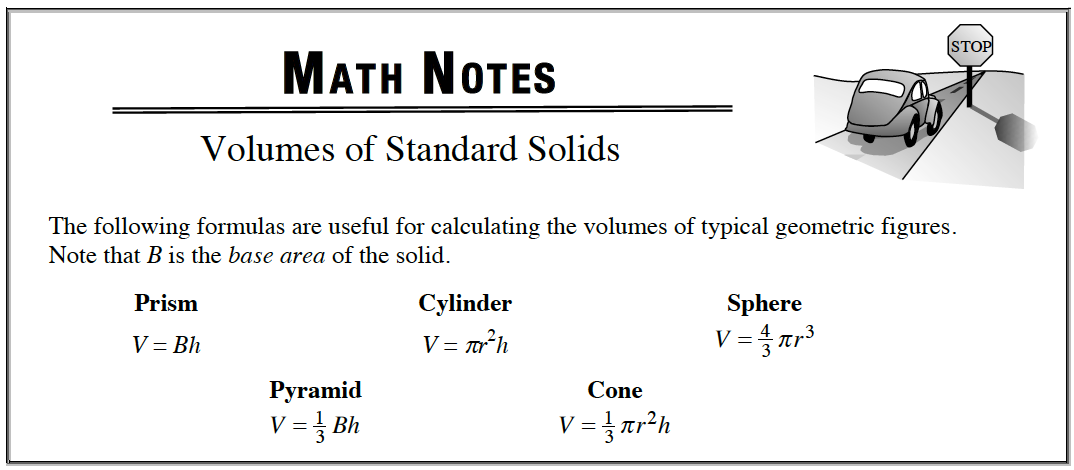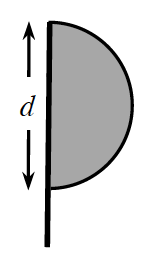### Home > APCALC > Chapter 1 > Lesson 1.2.3 > Problem1-60

1-60.

When the semi-circular flag below is rotated, it has a volume of $\frac { 243 } { 2 }$π un3Homework Help ✎

1. Describe the resulting three-dimensional figure.

Use the eTool below to visualize the rotating flag.
Click the link at right for the full version of the eTool: Calc 1-60 HW eTool

2. What is the value of $d$?

$\frac{243}{2}\pi =\frac{4}{3}\pi r^{3}$

$r=\frac{1}{2}d$3. If the diagram is rotated 90º and the flag is then rotated about a horizontal pole, will the volume change?

Build a model by taping a paper flag to a pencil. Try holding the pencil in different positions. Does the size or shape of the resultant solid change when the pencil is held horizontally vs. vertically?